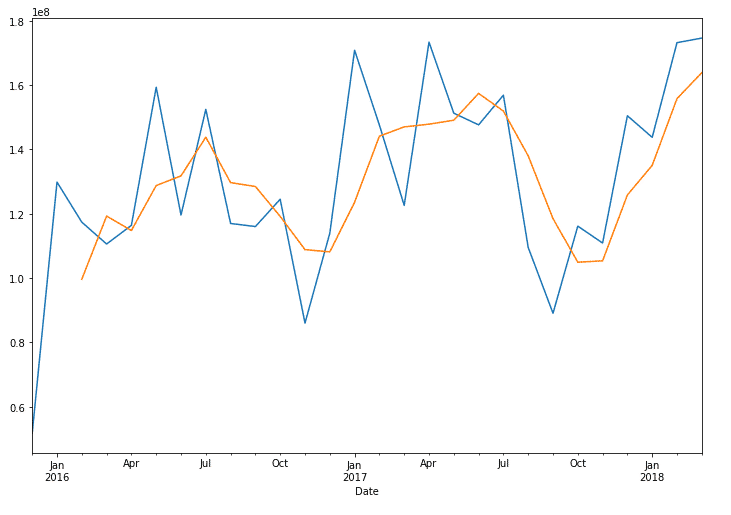# Python Moving Average

Creating a moving average is a fundamental part of data analysis. You can easily create moving averages with Python data manipulation package. Pandas has a great function that will allow you to quickly produce a moving average based on the window you define. This window can be defined by the periods or the rows of data.

Pandas ROLLING() function:

The rolling function allows you aggregate over a defined number of rows. If your rows are based on a day level of granularity, you will be aggregating over the day levels.

There is a window parameter to allow you to specify the span of the your aggregation.  Here is an example of rolling average for a 3 day window average if your data is at the day granularity.

ROLLING(WINDOW=3).MEAN()

Applying this function to a column would look similar to the below:

df[‘Column’].rolling(window=3).mean()

Let’s look at an example of this using our avocado dataset

Requirements

• datetime index

These function works off datetime index. So, you will need to setup the data below:

#load to the depenciesimport pandas as pddf = pd.read_csv(‘avocado.csv’,index_col=’Date’,parse_dates=True)%matplotlib inline#Limit the datas set and aggregate the to monthly. This is not necessary but for this data set it easier to visualize.df.head()df =df.loc[‘2015-12-12’:]df = df.resample(‘M’).sum()#create and visualize the rolling averagedf[‘Total Bags’].plot()df[‘Total Bags’].rolling(window=3).mean().plot()## Watch the Video.

• in
• |
• April 4, 2020#### Gaelim Holland

Subscribe
Notify of